### A thin film of soap will shine with all the colours of the rainbow. But why?

WATCH VIDEO:

Teachable Topics:

• Visible spectrum
• Snell's law
• Transmission & refraction

Theory:

A soap film has a thickness L and an index of refraction n. Light is incident on the film. The wavelength of light in air is l0. When an incident ray of light strikes the film, some of it is reflected (the reflected ray r1) and some of it is transmitted (the transmitted ray r2). What happens to each ray?

The reflected ray: Since n > nair, the index of refraction of air, when the light ray r1 reflects off the surface of the soap, its phase is shifted by l0/2.

The transmitted ray: The ray r2 travels through the soap, reflects off the back of the film at the soap-air interface, then travels back through the soap. At the first interface again, some of r2 is transmitted out to the air where it can interfere with r1.

Whether r1 and r2 interfere constructively or destructively depends on their relative phase difference when they meet again. We know that r1 is shifted by l0/2 when it reflects... now it remains to find the shift of r2.

Ray r2 travels a distance of 2L through a medium of index of refraction n before it meets r1 again. Its wavelength in this medium is l = l0/n, (according to a relation that is derived in an introductory physics text).

If r1 and r2 are to interfere constructively, r2 must have also been phase-shifted by a half-wavelength (l/2), or by l/2 + kl0 (where k = 1, 2, 3,.... an integer). This means that the distance 2L has to equal l/2 + kl.

2L = l/2 + kl

2L = l(k + 1/2)

2L = l0/n (k + 1/2)

If L = l0/2n (k + 1/2), then r1 and r2 interfere constructively, and the film appears bright.

If r1 and r2 are to interfere destructively, then r2 must be either unchanged or phase-shifted by an integral number of wavelengths. This means that the distance 2L the wave must travel has to equal a distance of kl.

2L = kl
2L = kl0/n

where k = 0, 1, 2, 3, ...

If L = kl0/2n, then r1 and r2 interfere destructively, and the film appears dark.

The incident ray is both reflected and transmitted at the air-soap interface. Ray r1 is phase-shifted one half wavelength when it is reflected. Ray r2 is phase-shifted by passing through a distance of 2L through the soap. Depending on the distance 2L, this can result in constructive interference...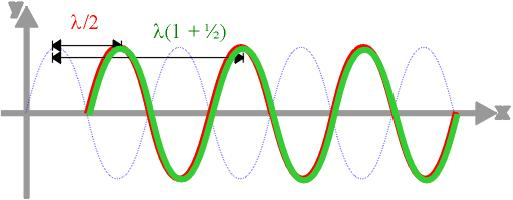...or in destructive interference...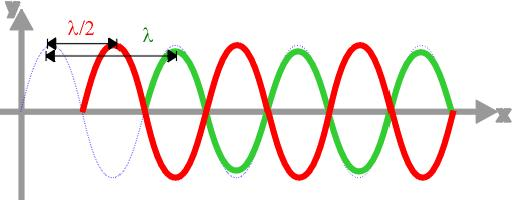An actual film of soap is usually not of uniform thickness, but has slight variations in thickness L. Because of this, if the film is lit with light of one wavelength (monochromatic light), l0, the film will have dark bands wherever the film thickness is L = kl0/2n
k = 0, 1, 2, 3, ...
Note that, in this formula, if k = 0, then L = 0. Of course we can't see interference in a thin film if there is no film there!!! However, if a film's thickness is considerably less than one wavelength of the incident light, then r1 and r2 will interfere destructively.

The film will have light bands where the thickness is

L = l0/2n (k + 1/2)

k = 0, 1, 2, 3, ... The most common illumination we experience is not monochromatic light, but white light, which is made up of a range of wavelengths. Each colour will have its bright areas and its dark areas on the film. This results in the appearance of the rainbow-colours on a thin soap film.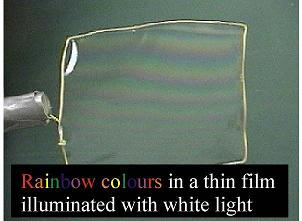Figure 1: Dispersion of white light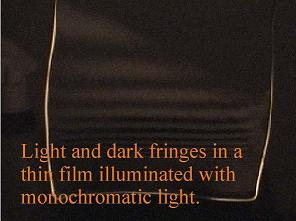Figure 2: Dispersion of monochromatic light

Apparatus:

• Water and soap mix
• Soap wand
• Source of white light
• Source of monochromatic light (e.g. a sodium lamp)

Procedure:

• Create a soap mixture by combining water and soap. As you stir the soap mixture, you will create lots of small bubbles at the surface of the liquid.
• Leave the soap solution out over night so the bubbles settle down. This will make creating soap films with the soap wand easier.
• To create a thin film, dip the wand into the solution and pull it out.
• Look at the soap film under white light and adjust your point of view until you can see the rainbow of colours. Note that the bands of colour are thinner near the bottom of the film. This happens because gravity makes the film bulge near the bottom, and the change in film thickness with respect to height is greater there.
• If a sodium light (or other semi-monochromatic light source) is available, repeat the above procedure using it. Using a white paper to diffusely reflect light from the source onto the film can help create "nicer" fringes.
• Using the monochromatic source one can see both light and dark fringes rather than the rainbow colours of white light.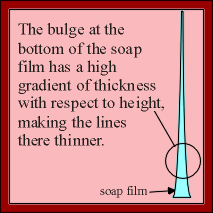Figure 3: Gravity's effect on film